# Evaluate 11

Multiply the quotient of 6 and 2 by 3, then add 1.

Add 1 and 6, then divide by 2 and multiply by 3.

Divide 6 by the product of 2 and 3, then add 1.

a =  10
b =  10.5
c =  2

### Step-by-step explanation: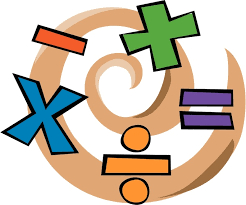Did you find an error or inaccuracy? Feel free to write us. Thank you!Tips to related online calculators
Do you want to perform natural numbers division - find the quotient and remainder?

## Related math problems and questions: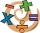Add six to twelve, then divide the sum by four. Divide the quotient by negative nine and then multiply by twenty-four. What is the result?
• DozenWhat is the product of 26 and 5? Write the answer in an Arabic numeral. Add up the digits. How many of this is in a dozen? Divide #114 by this
• Quotient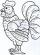Determine the quotient (q) and the remainder (r) from division numbers 100 and 8. Take the test of correctness.
• Quotient and productIf the quotient of [8/5 divided by 8/10] is added to the product of [8/14 x 7/12 x 3/8], what is the sum?Add marks (+, -, *, /, brackets) to fullfill equations 1 3 6 5 = 10 This is for the 4th grade of the primary school - with no negative numbers yet
• Evaluate - order of opsEvaluate the expression: 32+2[5×(24-6)]-48÷24 Pay attention to the order of operation including integers
• Expression plus minusEvaluate expression: (-1)2 . 12 – 6 : 3 + (-3) . (-2) + 22 – (-3) . 2
• Evaluate expressionIf x=2, y=-5 and z=3 what is the value of x-2y
• Expression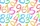If A=2 B=3 evaluate expression A(B+A) and multiply it by A
• Expression 8Evaluate this expressions: a) 5[3 + 4(2.8 - 3)] b) 5×(8-4)÷4-2
• Quotient 3If the quotient of 8/13 and 2 is subtracted from the product of 1 3/4 and 8/21, what is the difference?
• Expression 6Evaluate expression: -6-2(4-8)-9
• Product of the sum and differenceCalculate the product of the sum and difference of numbers -7 and -2.
• Evaluate valueEvaluate value of the following expressions: 1. The product of nine and a number 12, decreased by six 2. Eight decreased by three times a number 2. 3. The quotient of twice a number 49 and seven.
• With bracket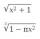Calculate (evaluate) simple mathematical expression with a negative numbers and a bracket: 13+15*5-2*(-6)
• Divide 11Divide the product of 4 and 5/8 by 1 1/2. Write your answer as a mixed number.
• What is 11What is the quotient of Three-fifths and 1 Over 10?